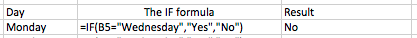# How to use the IF function in Excel to calculate values based on different criteria

The IF statement is a simple function in Excel that is one of the building blocks you need when you are working with large spreadsheets. You may not know you need it yet, but once you know how to use it, you won't want to live without it.

## IF() Function Syntax

The IF() function has the following syntax:

=IF(logical test, value if true, value if false)

The IF function works by performing a logical test that can only have one of two outcomes - TRUE or FALSE. It then outputs a result based on the outcome of that test.

## Logical Tests in Excel

You can use anything as a logical test provided Excel can determine whether the outcome is TRUE or FALSE.

Some examples of logical tests that you can use with the IF function include:

• C5=C6 (compare cell C5 to C6. If they are equal, the outcome is TRUE, otherwise the outcome is FALSE).
• C5>C6 (if C5 is greater then TRUE, otherwise FALSE)
• C5="" (if cell C5 is empty then TRUE, otherwise FALSE)
• SUM(A5:A10)>B5 (if the sum of cells A5 to A10 is greater than B5 then TRUE, otherwise FALSE).

There are a number of ways to construct more complex logical tests which we won't cover here.

## Examples of the IF() function in action

To use the IF() function, follow these steps:

• Click on a cell and enter the IF() function:• Enter the logical test as shown in this example:• The value in the Result column is the outcome of the IF formula
• The logical test checks to see whether the cell in the Day column (B5) = "Wednesday" (we use the speech marks to tell Excel the value we're performing the test on is text rather than a number)
• If the value in the Day cell is "Wednesday", then the result will be Yes.
• Otherwise the result will be No, as it is in this case.

There are many ways to use the IF() function in Excel that we won't cover here, but look out for more advanced IF() lessons coming soon.

## Our Comment Policy.

Add a comment to this lesson

### Adding cells that must be blank when no data present.

Hi, How do you add a formula to a cell that will only give a result when data is present, but will be blank when no data has been entered.
Then add more of these formatted cells together and again only show a result when some data has been entered, but remain blank when no data entered. I keep getting #value!

### Blank Cells referenced in my IF statement.

My IF statement is comparing the text value in two cells but I want the return to be blank if one of the cells is blank.
=IF(L2=N2, "Valid", "Variance")
However, if either L2 or N2 is empty, I don't want anything as the result.

### Hello

=IF(L2="",,IF(N2="",,IF(L2=N2, "Valid","Variance")))

It's called a nested conditional. There used to be a limit on how many levels a nest can go. I'm not sure if the limit is still there and how deep you can go.

### Using multiple IF statements in a single formula

Thanks Chris.

You can read about nested conditions in our lesson on Using multiple IF statements in Excel.

Regards

David

### IF THEN statements

IF(H16=2,1.5,IF(H16=1,1)
IF(H18=2 I need it to factor in 1.5-.5 how do I do that

### Using IF statement in Excel

If we use more than two cells to compare the value (Eg. "C5=C6=C7") den the result generated is false. Please suggest me the correct method.

### Can't Figure out error

I am working on a spreadsheet that requires multiple IF statements. It was working great until I had to add in 1 more variable and now there is an error and I cannot figure out what it is. Can someone help me out? Below is the formula:
=if(o12="m",(round(g12*01),2)),if(o12="y",(if(12=0,0,round(if(and(C\$5,c\$6,G12>f12),f12*.04,if(and(c\$5.c\$6,g12>f12),f12*.0275,f12*.01)),2),"NA")

I have also attached a copy

### John

mometasone spray over counter efeefafdbffegefb

### if FALSE calculate

This is wrong - and I know it... (I keep dragging my brain back to BASIC - yeah, I'm that old)

=IF(N3,0,"=D3*.0025")

the first half is right - if there is something in that field I do want this field to be 0.
However, if there is nothing in the first field I want excel to calculate D3*.0025...
and running down the sheet, D4*.0025; D5*.0025; etc...

Does anyone know the formula that will run the calculation?

### I hope you have resolved this

I hope you have resolved this by now, but for anyone who was googling to solve this exact issues, I discovered that you need to remove the quotations to get it register the cell recall
=IF(N3,0,"=D3*.0025")

becomes
=IF(N3,0,=D3*.0025)
the comma segments the formula, when using the quotation marks for the formula it will as a string of text between the quotes
"=D3*.0025"
returns as the text: "=D3*.0025